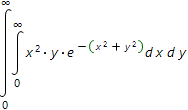# Double definite integrals online calculatorThe double integral computed over some domain, such as domain S, shown in the picture:The double integral over specified domain is calculated by reducing it to the repeated integral:The repeated integral consists of two simple definite integrals, for each of which, based on analysis of domain S, the limits of integration are obtained. In our case the first of the integrals is calculated by the variable y, and then, the result is integrated by the variable x.

Thus, in order to calculate double definite integral it is necessary to draw a region S and correctly define the limits of integration.

Our online calculator, based on Wolfram Alpha system, can only calculate the repeated integrals and it is your task to determine the limits of integration.

Double definite integrals calculatorInput the expression which double definite integral you want to calculate: